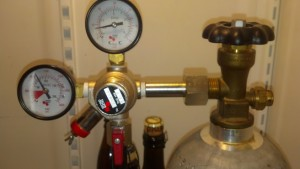# How to Calculate Beer Line Length

Today I’m going to talk about matching your line length and pressure on your home brewed keg. Trying to get your keg to pour nicely can be a real headache sometimes. But there is hope. There is an equation out there to calculate the right length, diameter and pressure to make your beers pour like a dream.

The equation is heavily based on what size or type of line you are using for your brew.  The size and type of your line determine the line’s resistance to let a fluid flow through it. There are many different lines used for draft beer and here are a few with their resistances.

3/16″ ID vinyl tubing = 3 psi per foot

1/4″ ID vinyl tubing = 0.85 psi per foot

3/16″ ID Polyethylene tubing = 2.2 psi per foot

1/4″ ID Polyethylene tubing = 0.5 psi per foot

You can see that the resistance follows a general trend, the bigger the line the less resistance. Keep in mind that you can’t go as small as you want, because eventually there will be too much resistance to move beer through the line and no amount of pressure will pour your beer. I wouldn’t go any smaller than 3/16” lines. So now that we have the resistance of our lines we can plug it and the other variables into the equation to determine your line length.

Line Length = (keg pressure – 1 psi)/Resistance

The minus one psi in the equation is to account for the fact that we want some pressure at the end of the line. Without that in the equation we would be solving for the pressure needed to reach exactly the end of the line.

So here is an example. If you are pressurizing your keg to 10 psi and you are using vinyl 1/4” tubing then the equation would spit out about 10.5 feet. Let’s say that is a lot of length that you don’t want it winding around all over the inside of your kegerator. So, we could adjust the pressure down to 5 psi. This would give us a length of 4.7 feet. This is a manageable length and we know that we should be getting a good pour from our keg if we use that length.

Even if you already have you line cut to 8 feet of 3/16th vinyl installed, we can calculate the best pressure for the keg. It’s just simple algebra.8 feet = (Keg pressure -1psi)/ 3

So 8*3 = Keg pressure – 1 psi

And  24+1 = keg pressure

Keg pressure = 25 Psi

What did we learn from that example? Don’t use 8 feet of 3/16 vinyl line to serve your beer or you have to keep the pressure very high.

One more equation deals with height. If your system delivers the beer, let’s say from a basement to the next floor you will need to account for the height. This equation works exactly the same way with one more variable, the height.

Line Length = (keg pressure – 1 – (height/2))/ Resistance

I trust that you can figure out this equation on your own and I hope this will help you pour better draft beer out of your keg. I know it has helped me.